# What is a timer ic

## Timers with the timer IC 555

Many timing circuits are usually easier to set up with operational amplifiers than with transistors. The most well-known integrated basic circuit is the timer IC 555. The standard version (NE 555) consists of 21 integrated bipolar transistors, some diodes and resistors. Comparable later types (LMC / TLC 555) are CMOS versions. Both versions are PIN-compatible and can also be used TTL-compatible in logic circuits with an operating voltage of 5 V. The 555 Timer IC can be used universally, as the properties of the various timing elements are only determined by the external circuitry. The following figure shows the functional units of the timer IC.

Block diagram of the timer IC 555

The operating voltage between pin 8 and pin 1 is divided by three equal resistors R (5 kΩ each) onto the reference voltages of the two comparators by two and one thirds. The higher voltage reference is brought out at pin 5. A 10 nF capacitor should be connected there to block short impulse interference. In addition, the reference values ​​can be influenced externally at pin 5.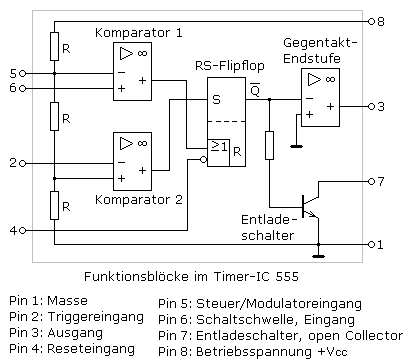The reset input of the RS flip-flop is actively connected to pin 4 of the timer IC by means of an OR gate low. This input must not remain open and must be connected to the operating voltage or a special reset control circuit. A low signal at pin 4 always leads to a reset of the RS flip-flop, regardless of other control signals. When switched on, the working state corresponds to a set RS flip-flop regardless of the external signal on pin 2.

The output signals of the two comparators control the RS flip-flop. If the potential at pin 6 exceeds the two-thirds reference, comparator 1 sets a high signal at the output. The RS flip-flop is reset by the internal reset. The Q-not output changes to the high state. The transistor as a discharge switch becomes conductive with low resistance. The output amplifier inverts the Q not high state and switches pin 3 to ground potential.

If the value falls below a third of the reference value at the trigger input pin 2, the comparator 2 switches its output to the high state and resets the RS flip-flop. Its Q-not output changes to the low state. Pin 7 goes high and pin 3 goes high.

Typical operating data

The timer ICs in its bipolar version works with operating voltages between 4.5 ... 15 V, with the SE 555 with a maximum of 18 V. The safe bandwidth extends up to 0.5 MHz. The output pin 3 can be switched as a current source or sink and switches a current of up to 100 mA. At 15 V operating voltage, a maximum of 200 mA is possible. The discharge switch on pin 7 can switch a maximum of 200 mA.

The CMOS version of the timer IC can also work with operating voltages below 4.5 V. The bandwidth at 15 V is higher and is typically 3 MHz. The output amplifier at pin 3 can withstand a maximum current load of 10 mA. The maximum discharge current at pin 7 is 100 mA.

### Bistable multivibrator

According to its name, a bistable multivibrator, also known as a flip-flop, has two defined stable switching states. A separate control signal is required for each state. The timer IC has an externally controllable RS flip-flop internally and can be used accordingly. The trigger input pin 2 works active low and forms the set input. A high signal at the threshold switch pin 6 resets the flip-flop. The following picture shows the timer IC as a bistable multivibrator. The circuit diagram shows how it works.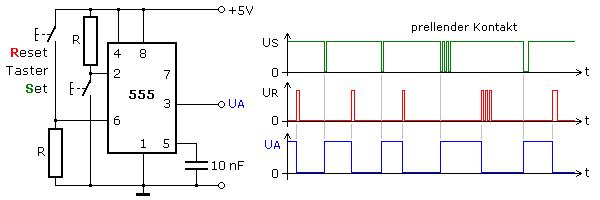When starting without control signals, the internal RS flip-flop is set and its Q-not output level is low. The output stage inverts the signal and output pin 3 starts with a high state. When the working state is set, the button on pin 2 has no effect.

The reset takes place when the button on pin 6 is pressed, which briefly connects the input to the operating voltage and thus switches comparator 1. The internal reset resets the RS flip-flop. Its Q-not output is set to high and the output pin 3 shows ground potential. The set and trigger input pin 2 is active low. The button briefly connects pin 2 to ground and sets the flip-flop again with the resulting high signal from comparator 2. The output signal at pin 3 switches to the high state.

Mechanical switching contacts tend to bounce and can cause switching malfunctions. The circuit diagrams show that similar pulse sequences no longer have any influence after switching the flip-flop into the stable state. Flip-flops are good for debouncing mechanical switches.

### Monostable multivibrator with the timer IC 555

The following figure shows the use of the timer IC and the switching behavior as a monostable multivibrator. Based on a potential on pin 2 that is greater than the one-third reference voltage on comparator 1, the RS flip-flop keeps the discharge switch on pin 7 conductive. The capacitor of the time-determining RC element remains discharged. The output at pin 3 has ground potential.

If the falling edge of the trigger signal at pin 2 falls below the value of one third of the reference voltage, the flip-flop is set in the timer IC. This state remains as long as the voltage at pin 2 is below the reference voltage. Therefore, the pulse duration must be shorter than that of the desired switching time of the monoflop. To ensure this, the trigger signal can be differentiated into needle pulses by a high pass at the input.After triggering, the output signal changes to the high state. The capacitor is charged from the operating voltage via the resistor R. The input pin 6 for the switching threshold is connected to the capacitor. A new triggering can take place at the earliest when the reference voltage is exceeded. This time T can be derived from the exponential function of the charging of the capacitor.

### Frequency divider

The monostable multivibrator shown above can be used as a frequency divider. It cannot be retriggered while the capacitor is charging. The RC element is calculated in such a way that the multivibrator resets itself within the trigger pulse to be divided. The subsequent trigger pulse generates a new output cycle. The following picture shows the pulse train.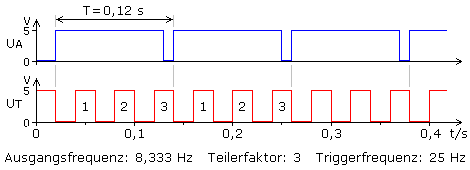### Astable multivibrator with the timer IC 555

With a small change in the circuit, the timer IC works as a square-wave generator with a fixed frequency. As long as the discharge switch on pin 7 is not conducting, the capacitor C is charged via the resistors R1 and R2. If the capacitor voltage reaches the two-thirds value of the supply voltage at pin 6, the RS flip-flop is switched over by comparator 1. The discharge switch conducts and is discharged through resistor R2. If the capacitor voltage falls below a third of the reference value on pin 2, comparator 2 resets the flip-flop. A new period of the square-wave signal begins at the output pin 3.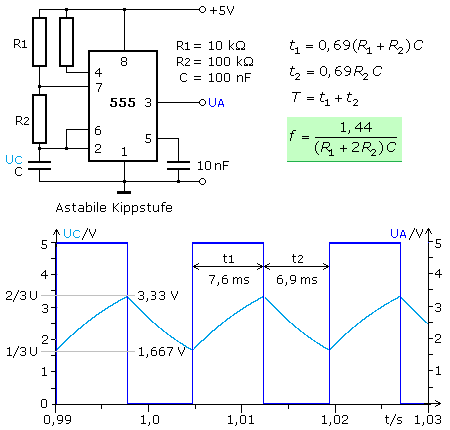The two switching times are always different because the resistor R1 cannot be arbitrarily small. Its lowest value is determined by the operating voltage and the maximum permissible discharge current of the timer IC. A circuit expansion with two diodes prevents this disadvantage. The following figure shows the astable multivibrator with equal resistances and an almost time-symmetrical square-wave signal.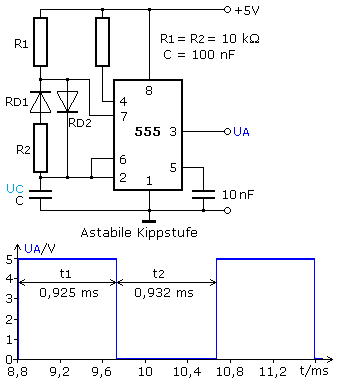The diode RD2 bridges the resistor R2 when it is conductive. The capacitor is only charged via R1. The discharge takes place via the series connection of R2 and RD1. If both resistors have the same value, the switching times should be identical according to the determining equations given above.

A mathematically exact match is not achieved because the dynamic resistance of the diodes in the conductive state cannot be neglected. For this reason, the frequency is also dependent on the operating voltage, which is higher at higher voltages. With the specified dimensions, the evaluation of the simulation at 5 V, 10 V and 15 V resulted in a square-wave frequency of 538 Hz, 630 Hz and 650 Hz.

If you use the discharge switch on pin 7 with a so-called pull-up resistor Rpu as an output and the change in output voltage on pin 3 to recharge the capacitor, an optimal time-symmetrical square-wave generator is created. The capacitor C1 is reloaded via the resistor R1 from the output amplifier at pin 3. The capacitor voltage controls the interconnected threshold and trigger inputs and switches the output of the RS flip-flop.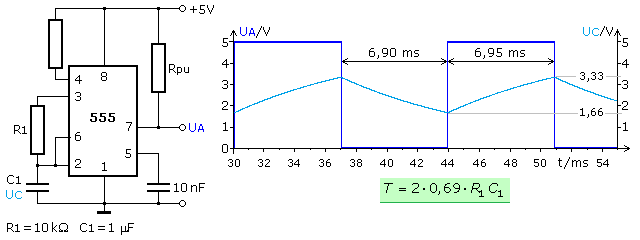The switching times of the square-wave signal are the same, since the charge reversal always takes place on the same RC element within the same one-third switching level. The square-wave signal has a low DC offset because the transistor switch cannot switch its collector-emitter voltage to exactly 0 volts when it is conductive. In the simulation the value was 50 mV. The CMOS version of the timer IC should work even better in this regard.

### Pulse width modulation with the timer IC 555

So far, the control or modulator input at pin 5 has not been used. The blocking capacitor switched to ground kept switching interferences to the internal voltage divider away from the reference voltages. If a control voltage is applied to this input, the reference voltages are influenced. The output signal of the astable multivibrator changes the frequency of the square-wave signal, the variation in the switch-on and switch-off times is more noticeable. The output signal corresponds to a pulse width modulation. The following circuit shows the working principle.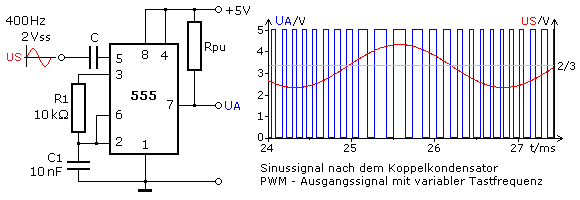If the pulse frequency of the PWM is an important criterion, the timer IC must be externally controlled with a suitable clock generator. If only voltage changes are to be recorded, the circuit principle shown fulfills this task. The voltage changes occurring at an external measuring resistor vary the switch-on and switch-off times of the timer output signal applied to pin 5. They can be used in a suitable control loop to counteract the veining.